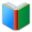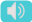# 2nd Grade Word List - Math

Words for students of 2nd Grade, the page is for math words (List 1), which are essential vocabulary for 2nd Grade academic classes.
 Free Online Vocabulary TestK12, SAT, GRE, IELTS, TOEFL
 Actions upon current list

 All lists of current grdae
Sight Words:

Arts Words:

Social Words:

Math Words:

Science Words:

Literature Words:The Real PrincessThe Old HouseThe Frog PrinceBriar RoseRapunzelThe Robber BridegroomKing Grisly-BeardLittle Red CapThe Sweetheart Roland
 centimetera metric unit of length equal to one hundredth of a meter
 congruentpossessing congruity; suitable; agreeing; corresponding
 equivalentbeing essentially equal to something
 evenoccurring at fixed intervals; not odd; capable of division by two without a remainder
 expanded notationshowing place value by multiplying each digit in a number by the appropriate power of 10
 Fahrenheitof or relating to a temperature scale proposed by the inventor of the mercury thermometer
 feeta unit of length in the U.S. Customary and British Imperial systems equal to 12 inches
 fourthone of four equal parts
 fractionsegment; fragment; a small proportion of
 growingrelating to or suitable for growth
 halfone of two divisions; consisting of one of two equivalent parts in value or quantity
 hundredsnumerical value equal to 100
 incha unit of measurement for advertising space; a unit of length equal to one twelfth of a foot
 meterthe basic unit of length adopted under
 notationtechnical system of symbols used to represent special things; comment or instruction
 oddnot easily explained; beyond from the usual or expected; not divisible by two
 operationa process or action, such as addition, substitution, transposition, or differentiation, performed in a specified sequence and in accordance with specific rules
 pictographa graphic character used in picture writing
 probabilitya measure of how likely it is that some event will occur; the quality of being probable
 repeatingthe act of doing or performing again
 rulermeasuring stick with a straight edge that is used for drawing straight lines and measuring lengths
 standardcriteria; basis for comparison
 standard-unitunits commonly encountered for a particular quantity
 symmetrichaving similarity in size, shape, and relative position of corresponding parts
 thirdfollowing the second position in an ordering or series; one of three equal parts of a divisible whole
 yardrod; stick; branch; long piece of timber; measure of length, equaling three feet
 Quick Access Worksheet: